# Partitive Division of Whole Numbers Revealing a Fractional Quotient

Visual number talk prompts that explore partitive division of whole numbers resulting in a fractional quotient (or fractional answer).

## In This Set of Math Visual Prompts…

Students will explore dividing whole numbers through partitive division to reveal a rate; some of which are whole quantities and some which are fractional quantities.

## Intentionality…

This set of visual number talk prompts is taken from the Number Talk section of Day 2 in the Make Math Moments Problem Based Unit called Snack Time! The purpose of this Visual Number Talk is to reinforce key concepts and big ideas from this problem based math unit including:

• Partitive division is one of the two structures of division;
• In partitive division, the dividend is the quota and the divisor is the number of parts or groups;
• When dividing partitively, the quotient reveals a rate, how many per part (or the quota per group);
• The quotient can be expressed as any fraction equivalent to the dividend over the divisor;
• The number of partitions determines the fraction unit and gives the quantity its name (i.e.: partitioning a whole into 5 parts creates “5 fifth” parts); and,
• Different denominators can be used to represent equivalent quantities.

Want to take a deeper dive into these concepts? Consider starting from the beginning of this 5-day problem based unit.

## Preparing to Facilitate This Number Talk

Present the visual number talk prompt by playing the video, pausing where indicated throughout.

The prompts shared with students throughout the video involve:

How could the cheese slices be cut to share evenly with the crackers shown? Convince your neighbour.

During the first prompt, students will be working with partitive division of whole numbers resulting in a whole number quotient, while in the second prompt, the dividend and divisor will swap resulting in a fractional quotient. Because this is partitive division in both cases, the resulting quotient is a rate (i.e.: how many cheese slices per cracker).

This number talk can be explored with an animated approach leveraging the visual number talk prompt videos below or in a low-tech fashion by orally sharing with students and writing on a vertical surface like a chalkboard or whiteboard. Regardless of which approach you choose, it is highly encouraged that the facilitator annotate student thinking on a vertical surface such as a chalkboard, whiteboard or projector so students feel their thinking in honoured and worth sharing.

Four (4) additional visual math talk prompts are shared in the Teacher Guide from Day 2 of the Snack Time problem based math unit

Visual Math Talk Prompt #1

Begin playing the video to share the first visual number talk prompt and be ready to pause the video to allow for think time.

The prompt for visual number talk prompt #1 is:

How could the 10 cheese slices be cut to share evenly with the 5 crackers? Convince your neighbour.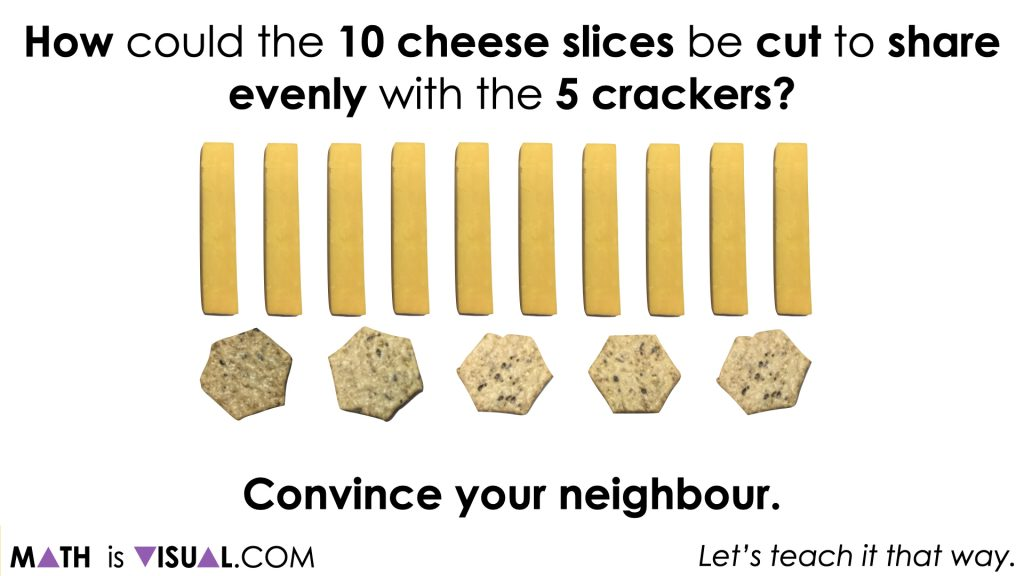With this first prompt, it is likely that many students can spatially partition the 10 slices of cheese into 5 groups or to fair share amongst the 5 crackers.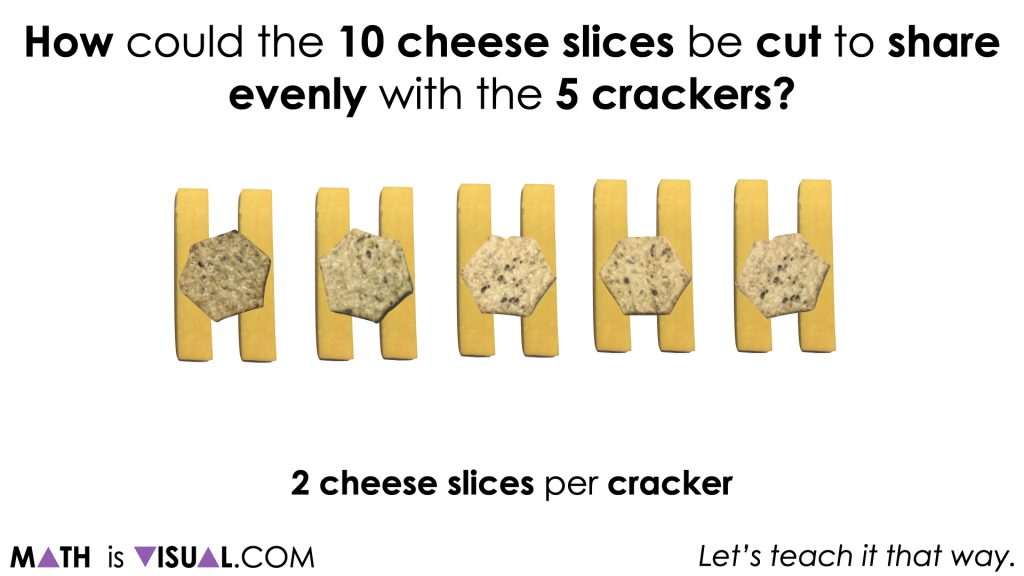Despite this feeling obvious to some (possibly many) students, be sure to ask students what operation is being used and to ask them to model this both visually and symbolically. One such visual model that can be helpful here is the double bar model or double number line which clearly shows the 2 to 1 ratio relationship that these quantities share.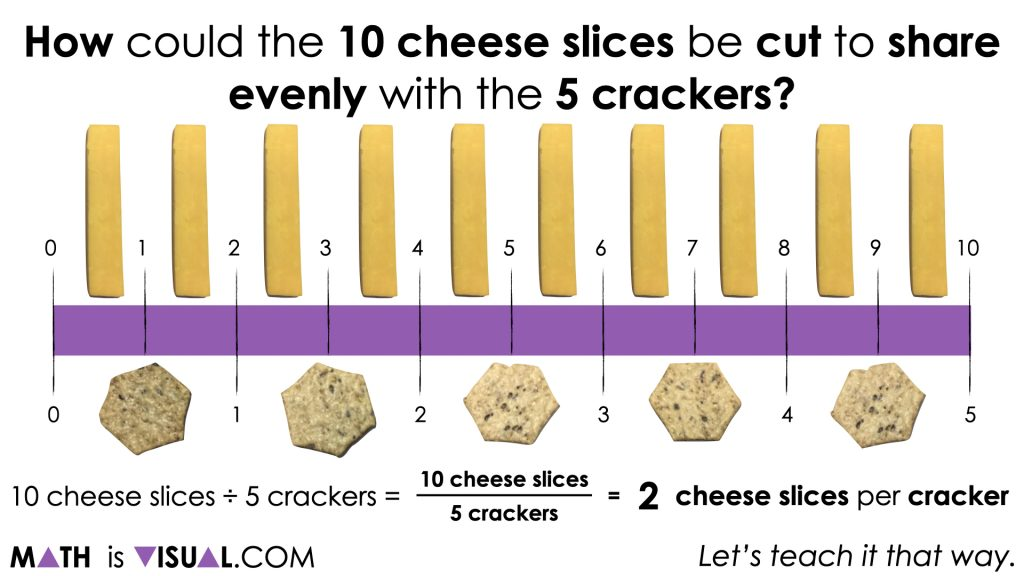Therefore, 10 cheese slices ÷ 5 crackers results in a rate of 2 cheese slices per cracker.

Visual Math Talk Prompt #2

Continue playing the video for the next Visual Math Talk Prompt.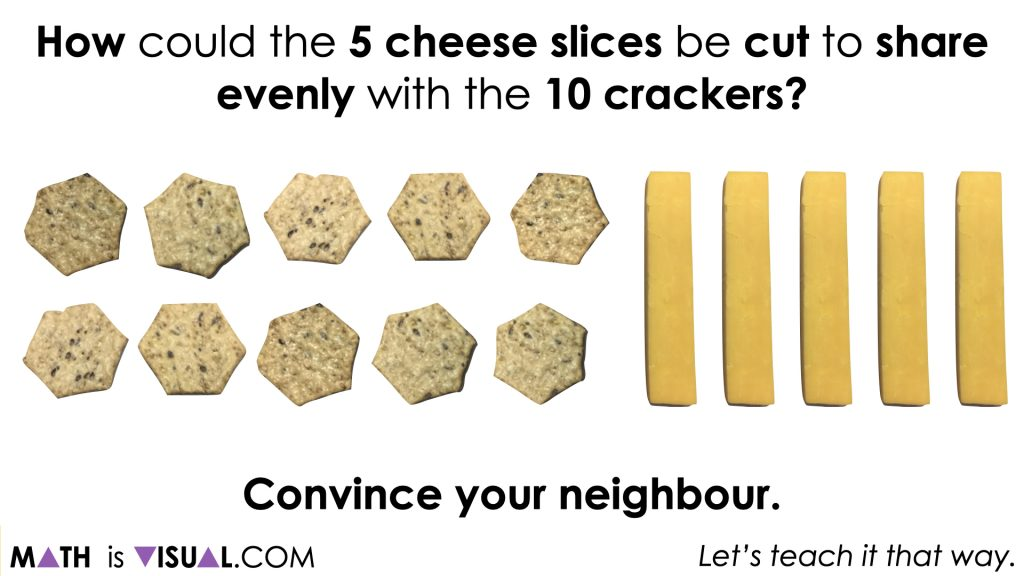While not as obvious as the result of the first visual number talk prompt, it is likely that students will realize that they can only fair share 1 full slice of cheese to half of the crackers and therefore must partition the cheese slices into halves (hence partitive division) to share an equivalent amount of cheese to each cracker.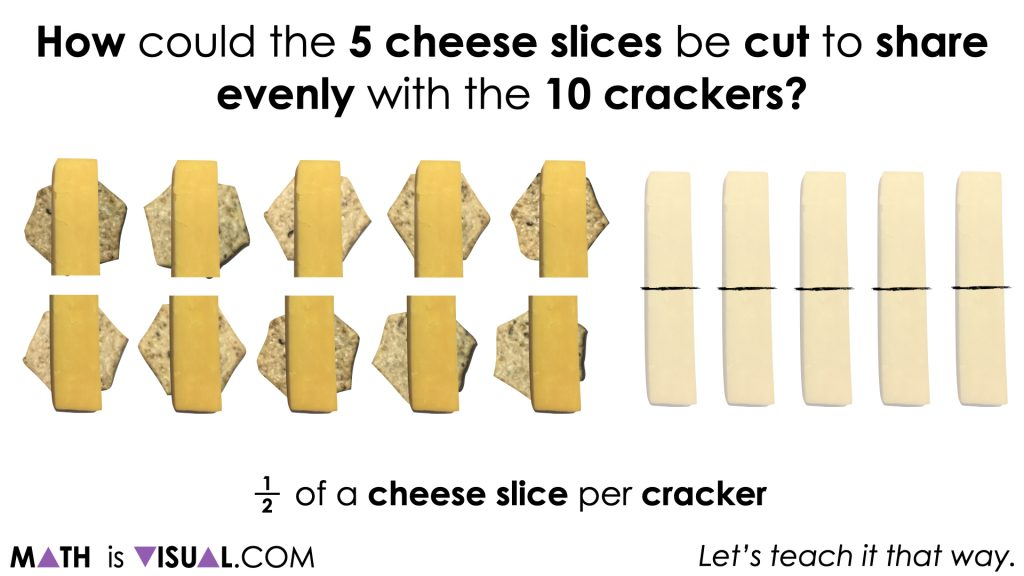While some students may model using a concrete or visual fair sharing approach as we see above, be sure to ask students to show what this might look like on a double bar model or double number line as well as symbolically.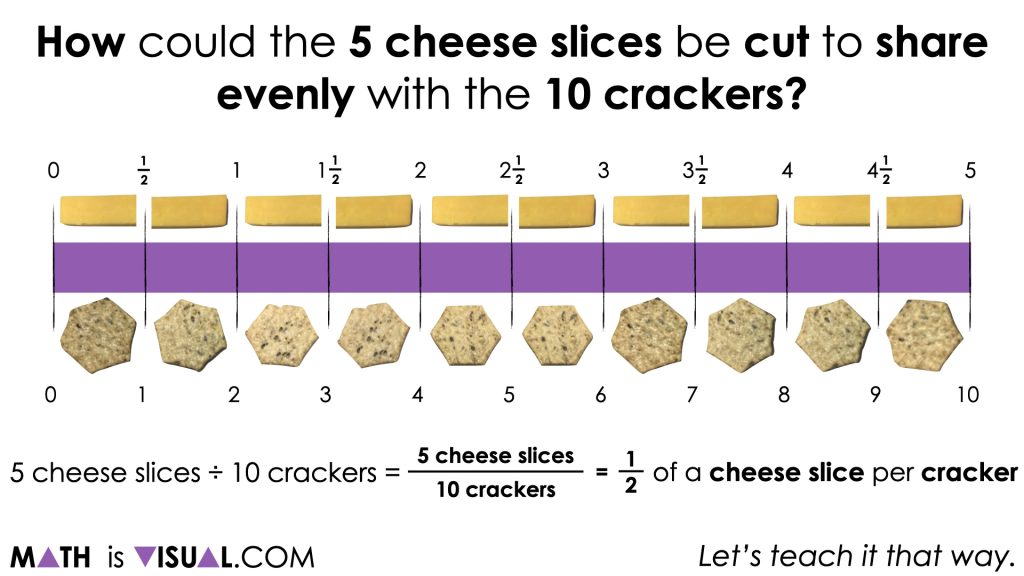Be sure to draw the attention of students to the 1 to 2 ratio of cheese slices to crackers and compare and contrast to the division sentence from the first visual number talk prompt where the ratio was the inverse.

## Want to Explore These Concepts & Skills Further?

Four (4) additional number talk prompts are available in Day 2 of the Snack Time problem based math unit that you can dive into now.

Why not start from the beginning of this contextual 5-day unit of real world lessons from the Make Math Moments Problem Based Units page.

Did you use this in your classroom or at home? How’d it go? Post in the comments!

Math IS Visual. Let’s teach it that way.